## Helmholtz Differential Equation--Spherical Coordinates

In Spherical Coordinates, the Scale Factors are,,, and the separation functions are,,, giving a Stäckel Determinant of. The Laplacian is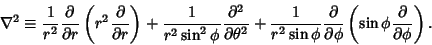(1)

To solve the Helmholtz Differential Equation in Spherical Coordinates, attempt Separation of Variables by writing(2)

Then the Helmholtz Differential Equation becomes(3)

Now divide by,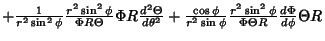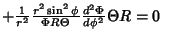(4)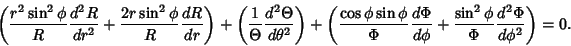(5)

The solution to the second part of (5) must be sinusoidal, so the differential equation is(6)

which has solutions which may be defined either as a Complex function with, ...,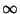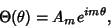(7)

or as a sum of Real sine and cosine functions with, ...,(8)

Plugging (6) back into (7),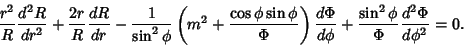(9)

The radial part must be equal to a constant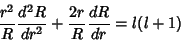(10)(11)

But this is the Euler Differential Equation, so we try a series solution of the form(12)

Then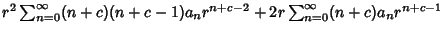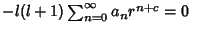(13)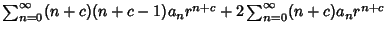(14)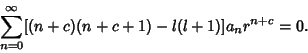(15)

This must hold true for all Powers of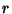. For theterm (with),(16)

which is true only ifand all other terms vanish. Sofor,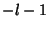. Therefore, the solution of thecomponent is given by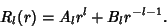(17)

Plugging (17) back into (9),(18)(19)

which is the associated Legendre Differential Equation forand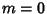, ...,. The general Complex solution is therefore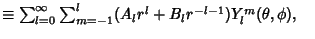(20)
where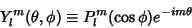(21)

are the (Complex) Spherical Harmonics. The general Real solution is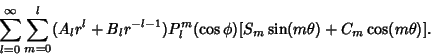(22)

Some of the normalization constants ofcan be absorbed byand, so this equation may appear in the form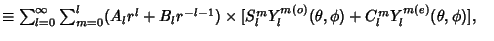(23)
where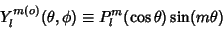(24)(25)

are the Even and Odd (real) Spherical Harmonics. If azimuthal symmetry is present, thenis constant and the solution of thecomponent is a Legendre Polynomial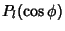. The general solution is then(26)

Actually, the equation is separable under the more general condition that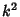is of the form(27)

References

Morse, P. M. and Feshbach, H. Methods of Theoretical Physics, Part I. New York: McGraw-Hill, p. 514 and 658, 1953.